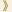HomeShaw Laureates2012Mathematical SciencesEssay

Traditionally the interaction between mathematics and theoretical physics has been concerned with topics ranging from dynamical systems and partial differential equations to differential geometry to probability theory. For the last two decades, modern algebra and algebraic geometry (which is the study of the solutions of systems of polynomial equations in several variables via algebraic methods) have taken a central position in this interaction.  Physical insights and intuition, especially from string theory, have led to a number of unexpected and striking predictions in both classical and modern algebraic geometry.  Thanks to the efforts of many mathematicians new techniques and theories have been developed and some of these conjectures have been proven.

Maxim Kontsevich has led the way in a number of these developments.  Among his many achievements is his early work on Witten’s conjecture concerning the topology and geometry of the moduli (that is parameter) spaces of all algebraic curves of a given genus, his solution of the problem of deformation quantization, his work in mirror symmetry and in a different direction the theory of motivic integration.

Quantization is the process of passing from classical to quantum mechanics and it has been realized by different mathematical theories.  One of these is the algebraic theory of deformation quantization.  This takes place on a Poisson manifold (that is a manifold with a Poisson bracket on functions) for which there are two natural algebras, the classical observables which are the functions under point-wise multiplication and the Poisson algebra where the multiplication comes from the Poisson structure.  The problem is to give a formal deformation in powers of a parameter h, in which the zeroth order term is the classical algebra of observables and the next order term is the given Poisson algebra.  The construction of such a deformation was carried out in special cases (Weyl, Moyal, Fedosov...) but the general case proved formidable. It was resolved brilliantly by Kontsevich using ideas from quantum field theory.

The discovery by physicists of mirror pairs of Calabi–Yau manifolds has led to a rich and evolving mathematical theory of mirror symmetry.  The physics predicts that there is a relation between the symplectic geometry (that is a geometry coming from classical mechanics) on such a manifold and the algebraic/complex geometry of the mirror manifold.  When carried out in certain examples for which explicit computations can be made, this led to some remarkable predictions in classical enumerative geometry, concerning the counting of curves in higher dimensional spaces.  Some of these predictions have since been proven. Kontsevich introduced homological mirror symmetry which predicts that further refined objects associated with the symplectic geometry of the manifold are related to ones associated with the complex geometry of its mirror.  These conjectures and their generalizations have been proven in significant special cases.  From the beginning Kontsevich has played a leading role in the development of the mathematical theory of mirror symmetry.  He continues to revisit the original formulation and to provide clearer conceptual answers to the mathematical question:   “What is mirror symmetry?”

Motivic integration is another invention of Kontsevich.  It is an integration theory which applies in the setting of algebraic geometry.  Unlike the usual integral from calculus whose value is a number, the motivic integral has its values in a large ring which is built out of the collection of all varieties (the zero sets of polynomial equations).  It satisfies many properties similar to the usual integral and while appearing to be quite abstract, when computed and compared in different settings it yields some far reaching information about algebraic varieties as well as their singularities.  It has been used to resolve some basic questions about invariants of Calabi–Yau varieties and it is also central to many recent developments concerning the uniform structure of counting points on varieties over finite fields and rings.

Through his technical brilliance in resolving central problems, his conceptual insights and very original ideas, Kontsevich has played a substantial role in shaping modern algebra, algebraic geometry and mathematical physics and especially the connections between them.

Mathematical Sciences Selection Committee
The Shaw Prize

17 September 2012, Hong Kong